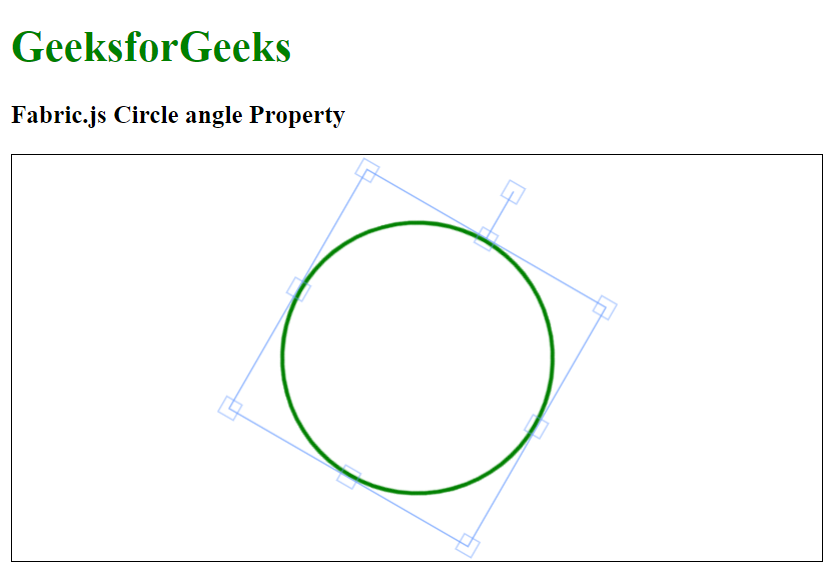# Fabric.js Circle angle Property

• Last Updated : 25 Jan, 2021

In this article, we are going to see how to draw a fixed angled canvas circle using FabricJS. The canvas circle is movable and can be stretched according to requirements. Further, the circle can be customized when it comes to initial stroke color, height, width, fill color, or stroke width.

To make this possible we are going to use a JavaScript library called FabricJS. After importing the library, we will create a canvas block in the body tag which will contain the circle. After this, we will initialize instances of canvas and circle provided by FabricJS and enable the angle of the canvas circle using angle property and render the circle on the canvas as given below.

Syntax:

```fabric.Circle({
angle: number
});```

Parameters: This function accepts two parameters as mentioned above and described below.

• angle: This parameter defines the angle of the circle.

Example: This example uses FabricJS to enable the angle of the canvas-like circle as given below. After enabling the angle property, it will rotate the circle by defined angle.

## HTML

 ```<``html``>`` ` `<``head``>``     ````    ``<``script` `src``=``"https://cdnjs.cloudflare.com/ajax/libs/fabric.js/3.6.2/fabric.min.js"``>``    `````` ` `<``body``>``    ``<``h1` `style``=``"color: green;"``>``        ``GeeksforGeeks``    ```` ` `    ``<``h3``>``        ``Fabric.js Circle angle Property``    ```` ` `    ``<``canvas` `id``=``"canvas"` `width``=``"600"` `height``=``"300"``        ``style``=``"border:1px solid #000000"``>``    ```` ` `    ``<``script``>`` ` `        ``// Initiate a Canvas instance ``        ``var canvas = new fabric.Canvas("canvas");`` ` `        ``// Initiate a Circle instance ``        ``var circle = new fabric.Circle({``            ``radius: 100,``            ``fill: '',``            ``stroke: 'green',``            ``strokeWidth: 3,``            ``angle: 30``        ``});`` ` `        ``// Render the circle in canvas ``        ``canvas.add(circle);``        ``canvas.centerObject(circle);``    `````` ` ``

Output:My Personal Notes arrow_drop_up